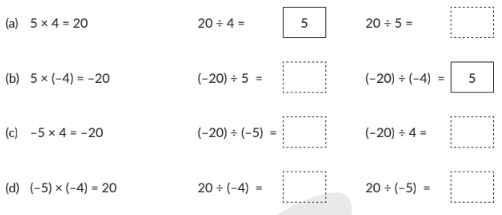In the verge of coronavirus pandemic, we are providing FREE access to our entire Online Curriculum to ensure Learning Doesn't STOP!

# Integers

Go back to  'Maths'

## Introduction to Integers

Taking the Positives with the Negatives!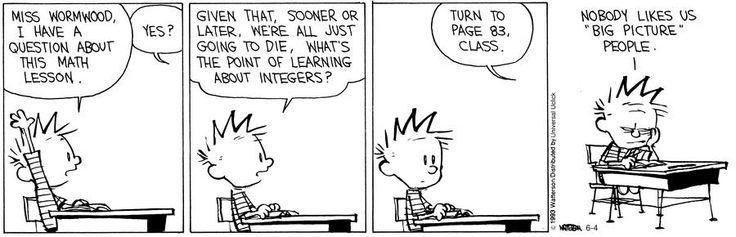Because integers are fun and really really helpful Calvin!

## What is an Integer?

An integer is a whole number that can be either positive or negative. Which means integers cannot be fractions and decimals (which you will learn later). Positive integers are basically the everyday numbers that we use to count things, for example ‘3 apples’, where 3 is a positive integer. As a result, positive integers are also sometimes called ‘counting numbers’. Negative integers are numbers that have a minus sign as a prefix, for example, -3 is a negative integer. Don’t worry they didn’t do anything wrong! Negative integers aren’t bad numbers! They just happen to be on the other side of the number line from positive integers!

### What is a Number line?

A number line is a picture of a straight line graduated with smaller lines to denote a number. At the centre of the number line is the number, zero. Since zero does not have a value associated with it, it is neither positive nor is it negative. The right-hand side of zero on the number line represents positive integers, whereas the left-hand side of zero represents the negative integers.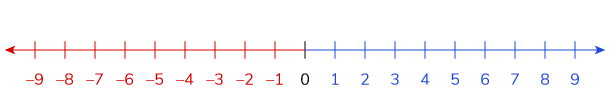Using the above number line, we can now familiarize ourselves with some basic operations of integers. Integers are important even in day to day life. You will often hear people say that the temperature has dropped by 5 degrees or that the prices of vegetables have gone up by 10 Rupees. The number line is useful for these situations because you can use it to visualize basic mathematical problems!

### Exercises with the Number Line

Before we dive into the world of integers, let’s familiarize ourselves with the number line. If you can visualize the number line, you’ll breeze through the chapters of integers in no time! Given below is a sample of a Cueamth worksheet from an integers book. Solve questions that are given. Draw a number line in your book to solve these questions. Take your time, there’s no hurry! Don’t let the wording of the problems confuse you! Before approaching each question, break it down to three steps:

Step 1: The starting point
Step 2: The change in the number line
Step 3: The ending point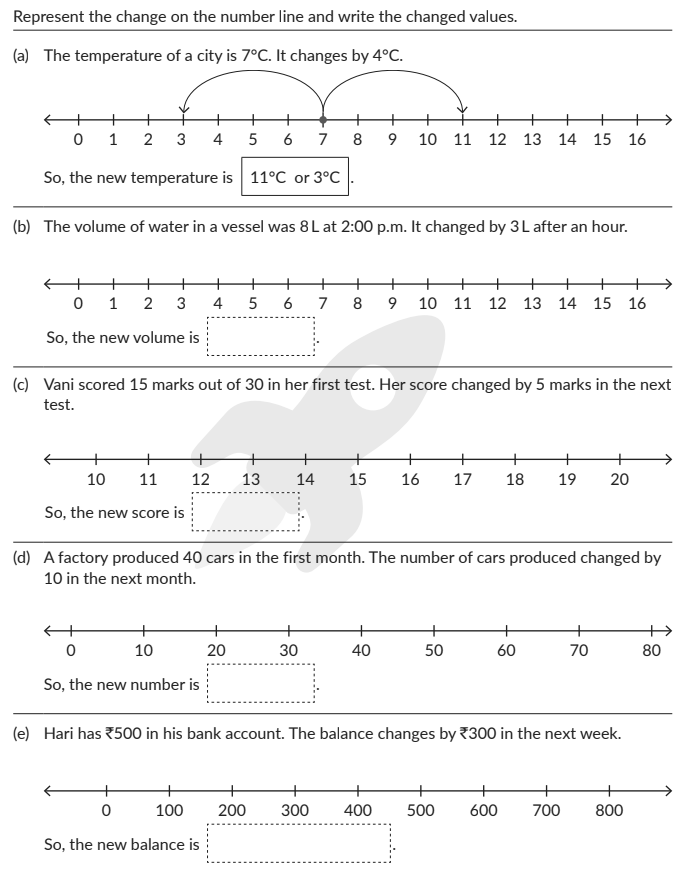## Topics Closely Related To Integers

The image shown below shows how different topics are connected to integers. What topics are required as a pre-requisite to master this topic? It also tells that mastery over integers can help build proficiency in connected topics like rational numbers.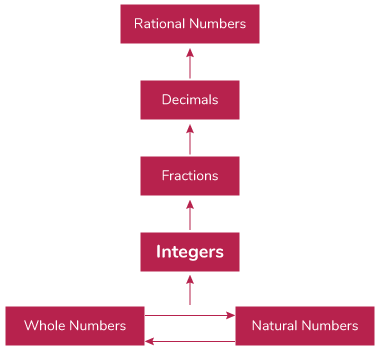## Sub Topics

Integers are a crucial step in your child’s mathematical journey. It is the stepping stone to fractions, decimals and eventually rational numbers. This is where you are first introduced to negative numbers and the negative end of the number line. Below topics cover both concepts and worksheets:

## Important concepts related to Integers

### The Absolute Value of an Integer

The absolute value of an integer is the numerical value of the integer without the negative or positive sign. On the number line, it is calculated as the distance between the number and zero.

For eg: the absolute value of -5 is 5. The absolute value of +5 is also 5.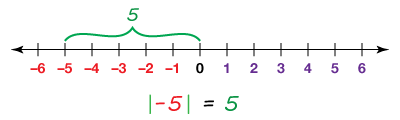### Addition and Subtraction of Integers

Now that we have the basics of integers completed, we can now move on to a few operations on integers. The easiest operations on integers are addition and subtraction. Before we dive into the complex problems, let’s solve some integer puzzles! These are some of the questions from the Cuemath workbook on integers.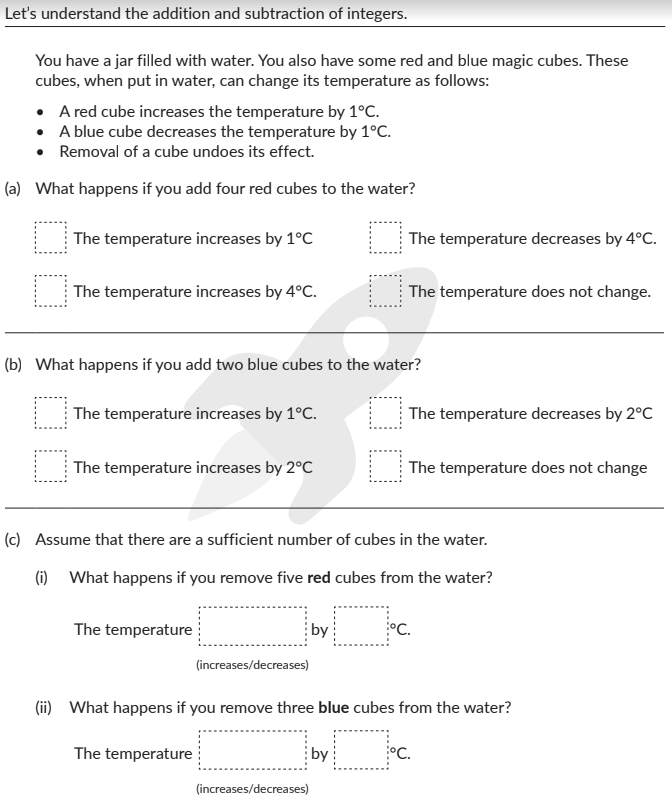Pretty simple right? When you add red cubes the temperature of the water increases by (1°C x number of red cubes). When you add blue cubes the temperature of the water decreases by (1°C x number of blue cubes). Okay, that was simple! For the next couple of problems, we are going to have the temperature of the water given to us. The trick to solving the problem is finding the difference between the original temperature of the water (given to us) and the final temperature of the water. Once you have done that subtraction, you can figure out which cubes were used red or blue, depending on the increase or decrease in temperature.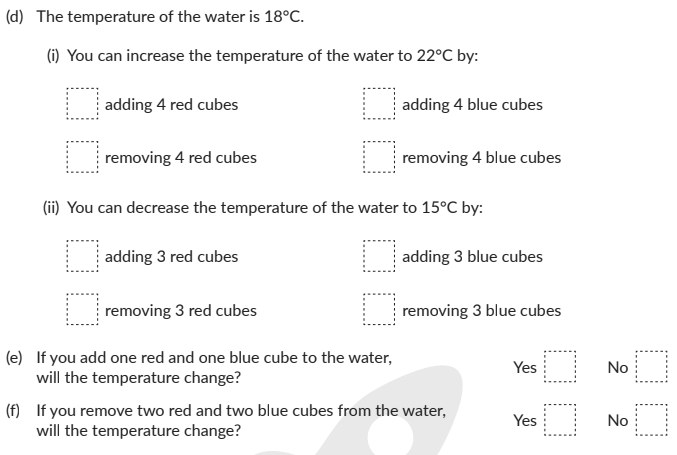Okay let’s take this puzzle to the next level, shall we? You must fill in the blanks with the appropriate integers in the dotted boxes. Remember that red cubes increase the temperature so they can be denoted by a '+' sign, and blue cubes decrease the temperature so they are denoted by a '-' sign. Here we go!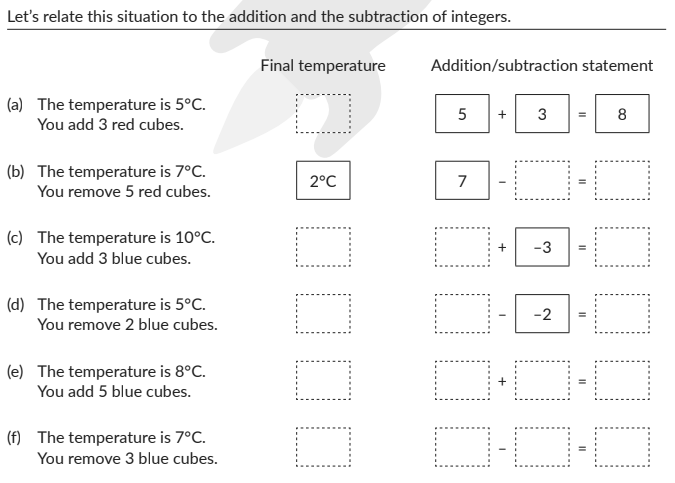At CueMath we believe that it is important for everyone to visualize their mathematical calculations! So again, we will use the number line to visualize addition and subtraction of integers. Remember, the more you can visualize integers the faster you’ll be able to add and subtract.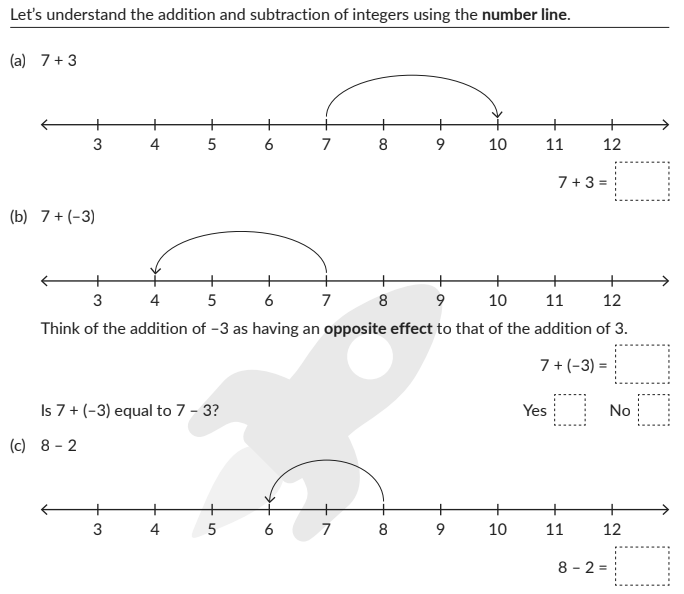### Multiplication and Division of Integers

Most adults don’t understand why signs change when you multiply a positive number with a negative number. Despite it being such an important lesson! So, let’s visualize this first and solve some problems!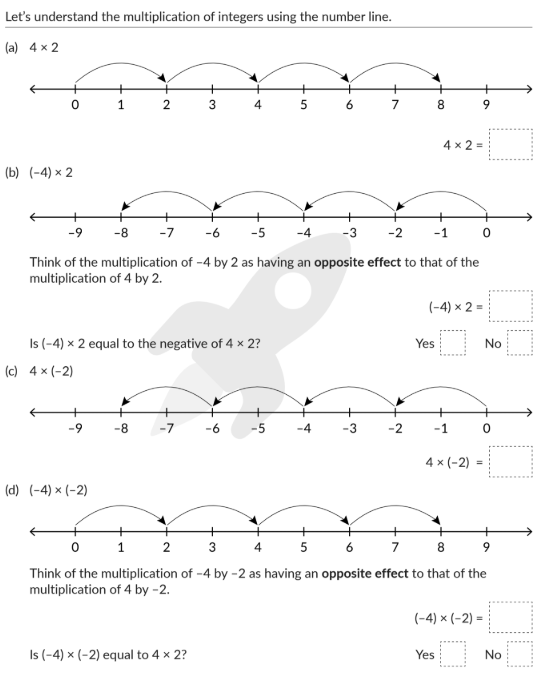Okay, now that we’ve learned how signs change during multiplication here are a few exercises for you to solve!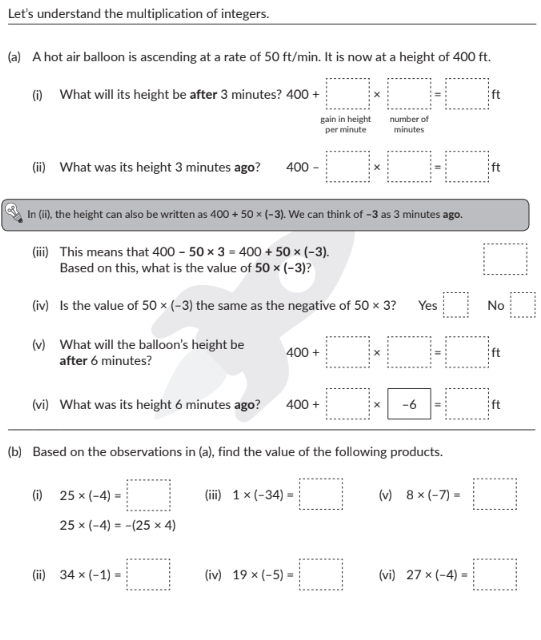The division of integers is the inverse process of their multiplication. Solve the following problems and write your answers on a piece of paper!